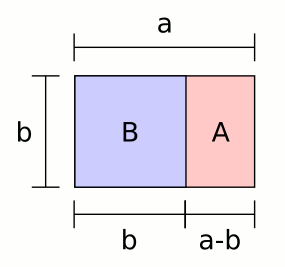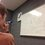# The Golden Ratio: What is it?Consider the above picture (called the golden rectangle). There is a rectangle that when we separate it into a square and another rectanlge, the rectangle formed is similar to the original rectangle. Let $a$ be the longer side of the rectangle, and $b$ the shorter side. Then, because of the rectangles' similarity, we have the proportion:

$\frac{a}{b} = \frac{b}{a-b}$

We will name this value $\phi = \frac{a}{b}$, the greek letter phi, and call it the Golden Ratio, just as the ancient Greeks did. At this point, this may just seem like some arbitrary geometrical figure. But $\phi$ has a lot of hidden properties, which we will uncover. First, let's try to find a numeric value for $\phi$: Taking the reciprocal of both sides of the equation above gives

$\frac{b}{a} = \frac{a-b}{b} = \frac{a}{b} - 1$

or, by definition of $\phi$,

$\frac{1}{\phi} = \phi -1$ $\rightarrow 0 = \phi^2 - \phi - 1$ $\rightarrow \phi = \frac{1+\sqrt{5}}{2} \approx 1.618$

Now we know the actual value of the ratio of the sides of the rectangle first pictured above. The following forms of equations involving $\phi$ will be very useful to us in the future:

$\phi^2 = 1 + \phi$ $\phi = 1 + \frac{1}{\phi}$

You may be wondering right now why I took only the positive root when I applied the quadratic formula to find $\phi$ above. In the geometric sense, I wanted a ratio, and ratios are always positive. The negative root results in a negative value for $\phi$. This value is called the conjugate of $\phi$ and has many similar properties to $\phi$. But we will normally restrict ourselves to the positive value of $\phi$. Tune in tomorrow for more mathematical information about the Golden Ratio.

Here is the next segment in the series.Note by Bob Krueger
7 years, 3 months ago

This discussion board is a place to discuss our Daily Challenges and the math and science related to those challenges. Explanations are more than just a solution — they should explain the steps and thinking strategies that you used to obtain the solution. Comments should further the discussion of math and science.

When posting on Brilliant:

• Use the emojis to react to an explanation, whether you're congratulating a job well done , or just really confused .
• Ask specific questions about the challenge or the steps in somebody's explanation. Well-posed questions can add a lot to the discussion, but posting "I don't understand!" doesn't help anyone.
• Try to contribute something new to the discussion, whether it is an extension, generalization or other idea related to the challenge.
• Stay on topic — we're all here to learn more about math and science, not to hear about your favorite get-rich-quick scheme or current world events.

MarkdownAppears as
*italics* or _italics_ italics
**bold** or __bold__ bold
- bulleted- list
• bulleted
• list
1. numbered2. list
1. numbered
2. list
Note: you must add a full line of space before and after lists for them to show up correctly
paragraph 1paragraph 2

paragraph 1

paragraph 2

[example link](https://brilliant.org)example link
> This is a quote
This is a quote
    # I indented these lines
# 4 spaces, and now they show
# up as a code block.

print "hello world"
# I indented these lines
# 4 spaces, and now they show
# up as a code block.

print "hello world"
MathAppears as
Remember to wrap math in $$ ... $$ or $ ... $ to ensure proper formatting.
2 \times 3 $2 \times 3$
2^{34} $2^{34}$
a_{i-1} $a_{i-1}$
\frac{2}{3} $\frac{2}{3}$
\sqrt{2} $\sqrt{2}$
\sum_{i=1}^3 $\sum_{i=1}^3$
\sin \theta $\sin \theta$
\boxed{123} $\boxed{123}$

## Comments

Sort by:

Top Newest

So, you're planning on doing posts everyday? Nice introductory post!

It should be noted that the golden-rectangle is said to be the most visually aesthetic rectangle.

- 7 years, 3 months ago

Log in to reply

Thanks! Every one or two days, that's correct. And about the golden rectangle being aesthetically pleasing, we will definitely be delving deeper into that concept! A quick estimation shows that this will probably continue through February.

- 7 years, 3 months ago

Log in to reply

Okay then! Good luck!

- 7 years, 3 months ago

Log in to reply

Nice, looking forward to more entries.

- 7 years, 3 months ago

Log in to reply

Good job! Keep it up, you still have a LOT to go through ;)

- 7 years, 3 months ago

Log in to reply

Thanks! I've already got the next six days written up.

- 7 years, 3 months ago

Log in to reply

nice work bro

- 7 years, 3 months ago

Log in to reply

Why are the two rectangles similar??

- 7 years, 1 month ago

Log in to reply

It's gr8...

- 7 years, 3 months ago

Log in to reply

I read first about this in THE DA VINCI CODE by Dan brown

- 7 years, 3 months ago

Log in to reply

nice work!

- 7 years, 3 months ago

Log in to reply

WOW! EXCELLENT.

- 7 years, 3 months ago

Log in to reply

It's great

- 7 years, 3 months ago

Log in to reply

×

Problem Loading...

Note Loading...

Set Loading...